### IMO Shortlist 1996 problem A9

Kvaliteta:
Avg: 0,0
Težina:
Avg: 10,0
Let the sequence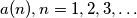$a(n), n = 1,2,3, \ldots$ be generated as follows with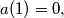$a(1) = 0,$ and for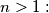$n > 1:$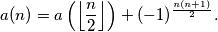1.) Determine the maximum and minimum value of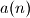$a(n)$ over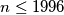$n \leq 1996$ and find all$n \leq 1996$ for which these extreme values are attained.

2.) How many terms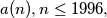$a(n), n \leq 1996,$ are equal to 0?
Izvor: Međunarodna matematička olimpijada, shortlist 1996